Courses
Courses for Kids
Free study material
Offline Centres
More

# NCERT Exemplar for Class 11 Physics Chapter 14 - Oscillations (Book Solutions)Last updated date: 08th Dec 2023
Total views: 612.6k
Views today: 14.12k

## NCERT Exemplar for Class 11 Physics - Oscillations - Free PDF Download

Free PDF download of NCERT Exemplar for Class 11 Physics Chapter 14 - Oscillations solved by expert Physics teachers on Vedantu  as per NCERT (CBSE) Book guidelines. All Chapter 14 - Oscillations exercise questions with solutions to help you to revise complete syllabus and score more marks in your examinations.

Oscillations in NCERT Exemplar Class 11 Physics  is a very important study material for CBSE Class 11 students. This Chapter covers the fundamental ideas needed to comprehend some of the topics covered in CBSE Class 12.

This NCERT exemplar Class 11 Physics  Chapter 14 PDF has essential questions on Oscillations, numerical on Oscillations, MCQs, Short and long answer questions, important questions from previous year question papers, and sample papers on Oscillations.

## Access NCERT Exemplar Solutions for Class 11 Physics Chapter 14 - Oscillations

### Multiple Choice Questions (MCQs)

14.1. The displacement of a particle is represented by the equation

$y = 3\cos \left( {\dfrac{\pi }{4} - 2\omega t} \right).$

The motion of the particle is

(a) Simple harmonic with period $\dfrac{{2\pi }}{\omega }$ .

(b) Simple harmonic with period $\dfrac{\pi }{\omega }$ .

(c) Periodic but not simple harmonic.

(d) Non-periodic.

Ans: Option (b)

When a force (called the restoring force) proportional to the displacement acts on a particle, it produces a simple harmonic motion. In nature, all sine and cosine functions of t are simple harmonics. As a result, the movement is simple harmonic motion. A simple harmonic motion is always periodic. Hence the motion is simple harmonic with time period  $\dfrac{\pi }{\omega }$.

14.2. The displacement of a particle is represented by the equation, $y = {\sin ^3}\omega t$ . The motion is

(a) Non-periodic.

(b) Periodic but not simple harmonic.

(c) Simple harmonic with period$\dfrac{{2\pi }}{\omega }$ .

(d) Simple harmonic with period $\dfrac{\pi }{\omega }$ .

Ans: Option (c)

All sine and cosine functions of t are simple harmonic in nature. Hence the motion is simple harmonic motion. A simple harmonic motion is always periodic.

Time period = $T' = \dfrac{{2\pi }}{\omega }$  Hence the motion is simple harmonic with time period.

This verifies the option (c).

14.3. The relation among acceleration and displacement of four Particles are given below.

(a) ${a_x} = + 2x$

(b)${a_x} = 2{x^2}$

(c) ${a_x} = - 2{x^2}$

(d)${a_x} = - 2x$

Which one of the particles is executing simple harmonic motion?

Ans: Option (d)

In simple harmonic motion acceleration is proportional and opposite to the displacement. Applying the equation of motion

$F = ma$

$a = - {\omega ^2}x$

$a$$\propto$$ - x$

Hence $- 2x$ represents SHM.

This verifies the option (d).

14.4. Motion of an oscillating liquid column in a U-tube is

(a) Periodic but not simple harmonic.

(b) Non-periodic.

(c) Simple harmonic and time period is independent of the density of the liquid.

(d) Simple harmonic and time-period is directly proportional to the density of the liquid.

Ans: Option (c)

Consider a U-tube filled with a dense liquid up to a height h, as illustrated in the diagram. When liquid is lifted from A to B in arm Q to a height of y From A' to C', the liquid level in arm P drops by y. 2y represents the height difference between the two arms.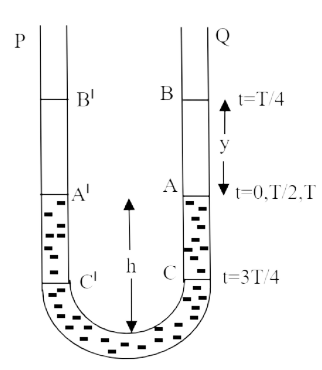Here the restoring force is provided by the hydrostatic pressure difference.

This is given by,

$F = - V.\rho .g = - A.2y.\rho g$

A= Area of cross section of tube

Then $F \propto - y$ , so motion is simple harmonic.

$T = 2\pi \sqrt {\dfrac{{m(inertia)}}{{k(spring)}}} = 2\pi \sqrt {\dfrac{{A(2h)\rho }}{{2A\rho g}}}$

$T = 2\pi \sqrt {\dfrac{h}{g}}$

Time period is independent of density and hence the motion is harmonic. So verifies the option (c).

14.5. A particle is acted simultaneously by mutually perpendicular simple harmonic motions ${\mathbf{x}} = {\mathbf{acos\omega t}}$ and${\mathbf{y}} = {\mathbf{asin\omega t}}$ . The trajectory of motion of the particle will be

(a) an ellipse.

(b) a parabola.

(c) a circle.

(d) a straight line.

Ans: Option (c)

Resultant displacement is given by $x + y$

$x = a\cos \omega t.......(1)$

$y = a\sin \omega t.......(2)$

Displacement = $a\cos \omega t + a\sin \omega t$

$y' = a(\cos \omega t + \sin \omega t)$

$y' = a\sqrt 2 \left[ {\dfrac{{\cos \omega t}}{{\sqrt 2 }} + \dfrac{{\sin \omega t}}{{\sqrt 2 }}} \right]$

$y' = a\sqrt 2 \left[ {\cos \omega t\cos {{45}^0} + \sin \omega t\sin {{45}^0}} \right]$ as the particle is acted simultaneously by mutually perpendicular direction.

$y' = a\sqrt 2 \cos s(\omega t - {45^0})$

Hence the displacement is neither a straight line nor a parabola.

Now, squaring and adding (i) and (ii)

${x^2} + {y^2} = {a^2}{\cos ^2}\omega t + {a^2}{\sin ^2}\omega t = {a^2}[{\cos ^2}\omega t + {\sin ^2}\omega t]$

${x^2} + {y^2} = {a^2}$

This is the equation of a circle, hence the motion is circular motion. This verifies option(c).

14.6. The displacement of a particle varies with time according to the relation${\mathbf{y}}{\text{ }} = {\text{ }}{\mathbf{asin\omega t}} + {\mathbf{bcos\omega t}}$ .

(a) The motion is oscillatory but not SHM .

(b) The motion is S.H.M. with amplitude  $(a + b)$ .

(c) The motion is S.H.M. with amplitude  $({a^2} + {b^2})$ .

(d) The motion is S.H.M. with amplitude$\sqrt {{a^2} + {b^2}}$ .

Ans: Option (d)

Given $y = a\sin \omega t + b\cos \omega t$

The amplitude of the motion is given by

$A = \sqrt {{a^2} + {b^2}}$

Hence verifies option (d)

14.7. Four pendulums A, B, C and D are suspended from the same elastic support as shown in Fig. 14.1. A and C are of the same length, while B is smaller than A and D is larger than A. If A is given a transverse displacement,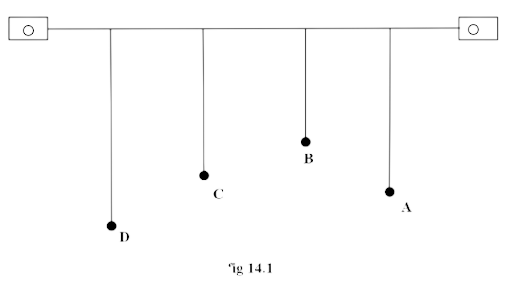(a) D will vibrate with maximum amplitude.

(b) C will vibrate with maximum amplitude.

(c) B will vibrate with maximum amplitude.

(d) All the four will oscillate with equal amplitude.

Ans: Option (b)

When the pendulum vibrates with transverse vibration then,

$T = 2\pi \sqrt {\dfrac{l}{g}}$ and $l$ = length of pendulum A and C.

The disturbance produce in elastic rigid support of time period T, which is transmitted by support to all pendulum B, C, D, but the frequency or time period of C is same as of A. So a periodic force of period T produces resonance in C and C will vibrate with maximum as in resonance. Hence, verifies the option (b).

14.8. Figure shows the circular motion of a particle. The radius of the circle, the period, sense of revolution and the initial position are indicated on the figure. The simple harmonic motion of the x-projection of the radius vector of the rotating particle P is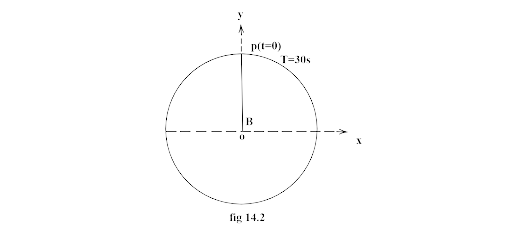(a)  $x(t) = B\sin \left( {\dfrac{{2\pi t}}{{30}}} \right)$

(b)  $x(t) = B\cos \left( {\dfrac{{\pi t}}{{15}}} \right)$

(c)$x(t) = B\sin \left( {\dfrac{{\pi t}}{{15}} + \dfrac{\pi }{2}} \right)$

(d)$x(t) = B\cos \left( {\dfrac{{\pi t}}{{15}} + \dfrac{\pi }{2}} \right)$

Ans: Option (a)

As the particle P is executing circular motion with radius B.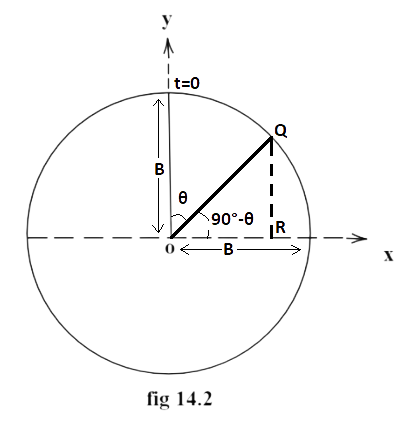Let particle P is at Q at instant t, foot of perpendicular on X-axis is at R vector OQ makes $< \theta$  with its zero Position not P displacement for O to R

$x = OQ\cos \left( {{{90}^0} - \theta } \right)$

$x = OQ\sin \theta = B\sin \omega t$   $\therefore \theta = \omega t$

$x = B\sin \dfrac{{2\pi }}{T}t$

$\therefore x = B\sin \left( {\dfrac{{2\pi }}{{30}}t} \right)$ hence this verifies option (a).

14.9. The equation of motion of a particle is $x = a\cos {(\alpha t)^2}$  . The motion is

(a) Periodic but not oscillatory.

(b) Periodic and oscillatory.

(c) Oscillatory but not periodic.

(d) Neither periodic or oscillatory.

Ans: Option (c)

Oscillatory but not periodic.

14.10. A particle executing S.H.M. has a maximum speed of $30cm/s$  and a maximum acceleration of  $60cm/{s^2}$ . The period of oscillation is

(a) $\pi \sec$

(b) $\dfrac{\pi }{2}\sec$

(c) $2\pi \sec$

(d)$\dfrac{\pi }{t}\sec$

Ans: Option (a)

Consider a SHM equation of motion

$y = a\sin \omega t$

$v = \dfrac{{dy}}{{dx}} = a\omega \cos \omega t...(i)$

$a = \dfrac{{dv}}{{dt}} = - a\omega \sin \omega t$

$a = - a{\omega ^2}\sin \omega t...(ii)$

${v_{\max }} = 30cm/s(given)$

$v{}_{\max }from(i)$

${v_{\max }} = a\omega$

$\therefore a\omega = 30...(iii)$

${a_{\max }} = a{\omega ^2}$   $from(ii)$

$60 = a{\omega ^2}....(iv)$  (Given)

$60 = \omega x30$

$\omega = 2rad/s$

$\dfrac{{2\pi }}{T} = 2$

$T = \pi$ , This verifies option (a).

### MULTIPLE CHOICE QUESTIONS-II MORE THAN ONE OPTION

14.11 When a mass is connected individually to two springs ${s_1}$and  ${s_2}$ , the oscillation frequencies are ${v_1}$ and ${v_2}$  . If the same mass is attached to the two springs as shown in Figure, the oscillation frequency would be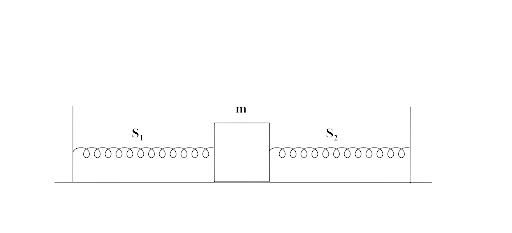(a)  ${v_1} + {v_2}$

(b) $\sqrt {{v_1}^2 + {v_2}^2}$

(c)  ${\left[ {\dfrac{1}{{{v_1}}} + \dfrac{1}{{{v_2}}}} \right]^{ - 1}}$

(d)$\sqrt {{v_1}^2 - {v_2}^2}$

Ans: Option (b)

When a mass (m) is connected to a spring on a horizontal frictionless surface then their frequencies are given by,

${V_1} = \dfrac{1}{{2\pi }}\sqrt {\dfrac{{{K_1}}}{m}} ..........(i)$  , and  ${V_2} = \dfrac{1}{{2\pi }}\sqrt {\dfrac{{{K_2}}}{m}}$

Now, the springs are in parallel because one end of both springs are connected with a rigid body and other end to body. So their equivalent spring constant becomes  ${k_p} = {K_1} + {K_2}$

And frequency ${v_p} = \dfrac{1}{{2\pi }}\sqrt {\dfrac{{{k_1} + {k_2}}}{m}}$

${v_p} = \dfrac{1}{{2\pi }}{\left[ {\dfrac{{{k_1}}}{m} + \dfrac{{{k_2}}}{m}} \right]^{\dfrac{{ - 1}}{2}}}$

From (i)   $\dfrac{{{K_1}}}{m} = {(2\pi {v_1})^2} = 4{\pi ^2}{v_1}^2$  and $\dfrac{{{k_2}}}{m} = 4{\pi ^2}{v_2}^2$

${v_p} = \dfrac{1}{{2\pi }}{\left[ {4{\pi ^2}{v_1}^2 + 4{\pi ^2}{v_2}^2} \right]^{1/2}}$ = $\dfrac{{2\pi }}{{2\pi }}{\left[ {{v_1}^2 + {v_2}^2} \right]^{1/2}}$

${v_p} = \sqrt {{v_1}^2 + {v_2}^2}$

Hence verifies option (b).

14.12. The rotation of earth about its axis is

(a) periodic motion.

(b) simple harmonic motion.

(c) periodic but not simple harmonic motion.

(d) non-periodic motion

Ans: Options (a) and (c)

The motion of earth about its own axis is circular motion and completes its one complete revolution in a regular interval of time. So the motion is said to be periodic. But motion is not about a fixed point from which we can measure its displacement or about which it moves both sides so it is not simple harmonic motion. So verifies the option (a) and (c).

14.13.  Motion of a ball bearing inside a smooth curved bowl, when released from a point slightly above the lower point is

(a) Simple harmonic motion.

(b) Non-periodic motion.

(c) Periodic motion.

(d) Periodic but not SHM

Ans: Options (a) and (b)

Let the ball be lifted from the point A to B inside the smooth bowl and released then it moves B to A then A to C, and come back to A from C and finally to B always, so motion is periodic just like a simple pendulum. Reaction force R (by blow) is balanced by $mg\sin \theta$ and a restoring force ($mg\sin \theta$) acts on the ball.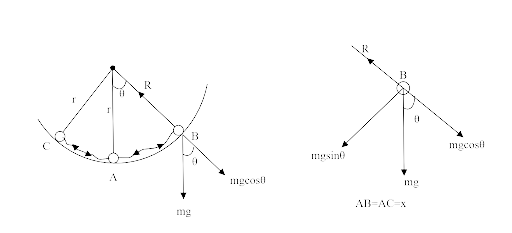So, $ma = mg\sin \theta$

$a = g\sin \theta$

Or $\dfrac{{{d^2}x}}{{d{t^2}}} = - g\sin \theta$ (When ball moves up)

$\dfrac{{{d^2}x}}{{d{t^2}}} = - g\dfrac{x}{r}$

(-) sign is because when ball moves upward displacement and force (or a) are opposite

$\therefore \dfrac{{{d^2}x}}{{d{t^2}}}$ $\propto$ $( - x)$ , so motion is SHM

$\omega = \sqrt {\dfrac{g}{r}}$ or $T = \dfrac{{2\pi }}{\omega } = 2\pi \sqrt {\dfrac{r}{g}}$

So motion is periodic and SHM. Hence verifies the options (a) and (c).

14.14.  Displacement vs. time curve for a particle executing S.H.M. is shown in Figure. Choose the correct statements.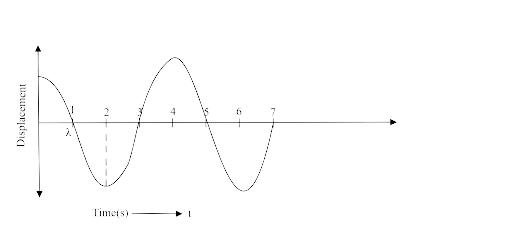(a) Phase of the oscillator is same at t =0 s and t = 2 s

(b) Phase of the oscillator is same at t=2s and t=6s

(c) Phase of the oscillator is same at t=1s and t=7s

(d) Phase of the oscillator is same at t=1s and t=5s

Ans: Options (b) and (d)

Two particles are said to be in same phase if the mode of vibration is same that is their distance will be  $n\lambda (n = 1,2,3,.....)$

(a) Distance between particles at t t=0 and t =2 is  $\dfrac{\lambda }{2}$

So, articles are not in the same phase.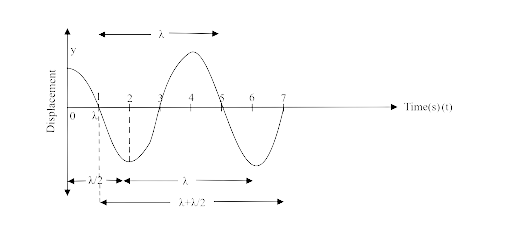(b) As from figure the particles at t = 2 sec and 6 sec are at distance $\lambda$  , so are in same phase

(c) Particles at t =1, t= 7 are the distance $\lambda + \dfrac{\lambda }{2} = \dfrac{{3\lambda }}{2}$  so are not in phase.

(d) Particles at t =1 sec and 5sec are at distance = $\lambda$  so are in the same phase. This verifies the options (b, d).

14.15. Which of the following statements is/are true for a simple harmonic oscillator?

(a) Force acting is directly proportional to displacement from the mean position and opposite to it.

(b) Motion is periodic.

(c) Acceleration of the oscillator is constant.

(d) The velocity is periodic.

Ans: Options (a), (b) and (d)

Consider a SHM  $x = a\sin \omega t - - - - - (i)$

$v = \dfrac{{dx}}{{dt}} = a\omega \cos \omega t - - - - - (ii)$

Acceleration $A = \dfrac{{dv}}{{dt}} = \dfrac{d}{{dt}}(a\omega \cos \omega t) = a\omega ( - \omega \sin \omega t)$

$\therefore A = - a{\omega ^2}\sin \omega t$ or $A = - {\omega ^2}x$

$mA = - m{\omega ^2}x$

$F \propto - (x)$

Hence, force is directly proportional to displacement opposite the direction of displacement. We can prove

$x(t) = x(t + T)$

So motion is periodic and SHM. From (ii) v is also periodic as we can prove

$v(t) = v(t + T)$

Hence, verifies the option.

14.16. The displacement time graph of a particle executing S.H.M. is shown in Figure. Which of the following statements is/are true?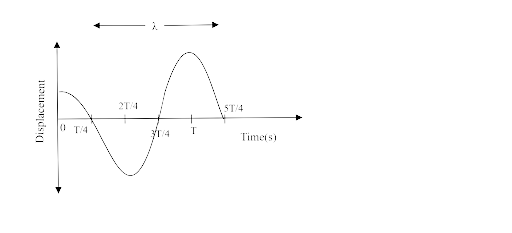(a) The force is zero at $t = \dfrac{{3T}}{4}$

(b) The acceleration is maximum at $t = \dfrac{{4T}}{4}$

(c) The velocity is maximum at $t = \dfrac{T}{4}$

(d) The P.E. is equal to K.E. of oscillation at $t = \dfrac{T}{2}$

Ans: Options (a), (b) and (c)

(a) At $t = \dfrac{{3T}}{4}$ Particle is at its mean position so force acting on it is zero, but it continue the motion due to inertia of mass, here a = 0, so F = 0.

(b) At $t = \dfrac{{4T}}{4} = T$ , particle’s velocity changes increasing to decreases so maximum change in velocity at T. As acceleration (Change in velocity)/ Time , so acceleration is maximum here.

(c) At $t = \dfrac{T}{4}$  it is at its mean position where the velocity is maximum with no retarding force on it.

(d) $t = \dfrac{T}{2} = \dfrac{{2T}}{4}$ , the particle has KE=0, So $KE \ne PE$  . Hence, verifies the option    (a, b, c).

14.17. A body is performing S.H.M. Then its

(a) Average total energy per cycle is equal to its maximum kinetic energy.

(b) Average kinetic energy per cycle is equal to half of its maximum kinetic energy.

(c) Mean velocity over a complete cycle is equal to $\dfrac{2}{\pi }$  times of its maximum velocity.

(d) Root mean square velocity is $\dfrac{1}{{\sqrt 2 }}$  times of its maximum velocity.

Ans: Options (a),(b) and (d)

(a) Let us consider a periodic SHM.

$x = a\sin \omega t$

Let mass m is executing the SHM

$v = \dfrac{{dx}}{{dt}} = a\omega \cos \omega t$

${v_{\max }} = a\omega (\because \cos \omega t = 1)$

$\therefore$ Total mechanical energy = $K.{E_{\max }}$ or $P.{E_{\max }}$

$\therefore T.E = \dfrac{1}{2}m{a^2}{\omega ^2}$

Or average Total energy is $K.{E_{\max }}$

$K.{E_{\max }} = \dfrac{1}{2}m{a^2}{\omega ^2}$

(b) If amplitude is a and angular frequency is $\omega$  , then maximum velocity of a particle will be equal to $a\omega$  and it varies according to Sine law. Hence, r.m.s. the value of velocity of the particle over a complete cycle will be equal to $- \dfrac{1}{{\sqrt 2 }}a\omega$ .

$\therefore$ Average K.E = $\dfrac{1}{2}m{v^2}_{rms}$

$- \dfrac{1}{2}m\left( {\dfrac{1}{{\sqrt 2 }}a\omega } \right) = \dfrac{1}{2}m\dfrac{1}{2}{a^2}{\omega ^2}$

$\therefore$Average K.E = $\dfrac{1}{2}\left\{ {\dfrac{1}{2}m{a^2}{\omega ^2}} \right\}$

$= \dfrac{1}{2}\left\{ {\dfrac{1}{2}{m^2}_{\max }} \right\}$       $\left[ {\because {v_{\max }} = a\omega } \right]$

$= \dfrac{1}{2}K.{E_{\max }}$

(c)  $v = a\omega \cos \omega t$

${v_{mean}} = \dfrac{{{v_{\max }} + {v_{\min }}}}{2} = \dfrac{{a\omega + ( - a\omega )}}{2}$

$v{}_{mean} = 0$  $\therefore$ ${v_{\max }} \ne {v_{mean}}$

(d) ${v_{rms}} = \sqrt {\dfrac{{{v_1}^2 + {v_2}^2}}{2}}$

$\Rightarrow \sqrt {\dfrac{{0 + {a^2}{\omega ^2}}}{2}} = \dfrac{{a\omega }}{{\sqrt 2 }}$

${v_{rms}} = \dfrac{{{v_{\max }}}}{{\sqrt 2 }}$

Hence, verifies the options (a, b, d).

14.18.(a) The sign of velocity, acceleration and force on the particle when it is 3 cm away from A going towards B are positive.

(b) The sign of velocity of the particle at C going towards B is negative.

(c) The sign of velocity, acceleration and force on the particle when it is 4 cm away from B going towards A are negative.

(d) The sign of acceleration and force on the particle when it is at point B is negative.

Ans: Options (a), (c) and (d)

(a) when the particle is going from A to B (+ direction) and it is 3 cm from A velocity increases up to O so velocity is positive.

Acceleration in SHM is towards  $+ ve$ . So both v and a are$+ ve$

(b) As the particle is going towards B so velocity is Positive not negative.

(c) As the particle is at 4 cm from B and B and going towards A ie, $+ ve$ side, so velocity and acceleration towards mean position at O. So both are negative.

(d) When a particle is at B force and acceleration both are towards ‘O’, so both are negative.

### VERY SHORT ANSWER TYPE QUESTIONS

14.19Displacement versus time curve for a particle executing S.H.M. is shown in Figure. Identify the points marked at which

(i) Velocity of the oscillator is zero.

Ans: Velocity of oscillator is maximum where the displacement is maximum i.e., at A, C, E and G.

(ii) Speed of the oscillator is maximum.

Ans: Speed of oscillator will be maximum when no restoring force acts i.e., where displacement of oscillator is zero. i.e., at B, D, F and H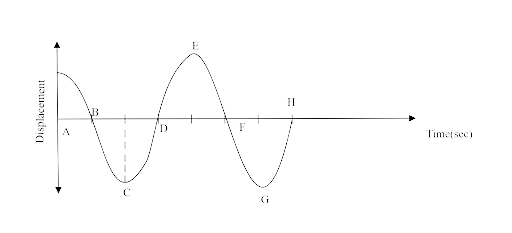14.20. Two identical springs of spring constant K are attached to a block of mass m and to fixed supports as shown in Figure. When the mass is displaced from equilibrium position by a distance x towards right, find the restoring force.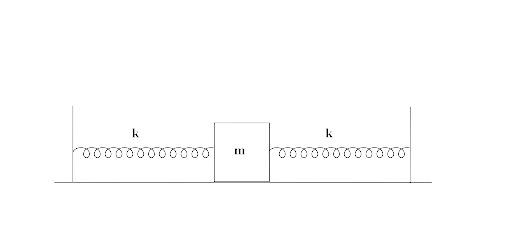Ans: When the mass m is displaced from equilibrium position by a distance x towards right then spring B will be compressed by distance x, and apply the force$(kx)$  to mass m towards left. But Spring A will extend by distance $x$  apply the force $(kx)$ on mass m towards left. But spring A will extend by distance x and apply the force $kx$ on mass towards left. So net force acting on the block towards the left side is restoring force.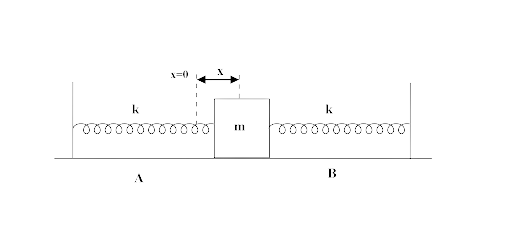$F = kx + kx = 2kx$

$F = 2kx$ (restoring force towards left) is the restoring force.

14.21. What are the two basic characteristics of a simple harmonic motion?

Ans: Two basic characteristics of a simple harmonic motion are:

(i) Acceleration is directly proportional to displacement from mean position, and the direction of acceleration is towards mean position.

(ii) Restoring force is directly proportional to displacement, the direction of force and displacement are opposite, that is $F = - kx$ .

14.22. When will the motion of a simple pendulum be simple harmonic?

Ans: Consider a pendulum of length l and mass of bob m is displaced by angle $\theta$  as shown in figure.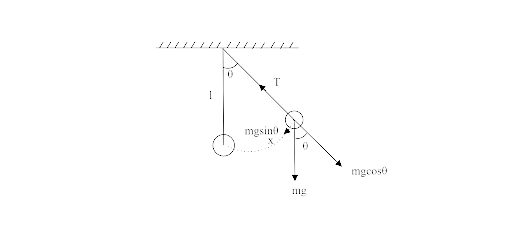The restoring force , $F = - mg\sin \theta$

If $\theta$  is small then $\sin \theta = \theta = \dfrac{{arc}}{{radius}}$

$\Rightarrow \dfrac{x}{l}$

$\therefore F = - mg\dfrac{x}{l}$  or $F\alpha ( - x)$

(m, g, l are constants)

Hence the motion of a simple pendulum will be harmonic for small angles $\theta$.

14.23. What is the ratio of maximum acceleration to the maximum velocity of a simple harmonic motion?

Ans: Consider a SHM , $x = A\sin \omega t$

$v = \dfrac{{dx}}{{dt}} = A\omega \cos \omega t$

For ${v_{\max }}$, $\cos \omega t = - 1$

$\therefore {v_{\max }} = A\omega$

$a = \dfrac{{dv}}{{dt}} = - A{\omega ^2}\sin \omega t$

For ${a_{\max }}$, $\sin \omega t = - 1$

${a_{\max }} = A{\omega ^2}$

$\therefore \dfrac{{{a_{\max }}}}{{{v_{\max }}}} = \dfrac{{A{\omega ^2}}}{{A\omega }} = \dfrac{\omega }{1}$

14.24. What is the ratio between the distance traveled by the oscillator in one time period and amplitude?

Ans: Distance traveled by oscillator in one time-period = 4A where A = amplitude of the oscillation.

Required ratio= $\dfrac{{4A}}{A} = \dfrac{4}{1}$

That is $4:1$

14.25. In Figure what will be the sign of the velocity of the point P′ which is the projection of the velocity of the reference particle P. P is moving in a circle of radius R in anticlockwise direction.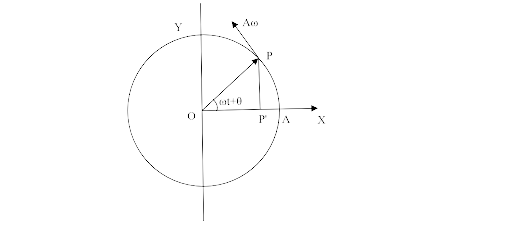Ans: $P'$  is the foot perpendicular to the velocity vector of particle P at any time t.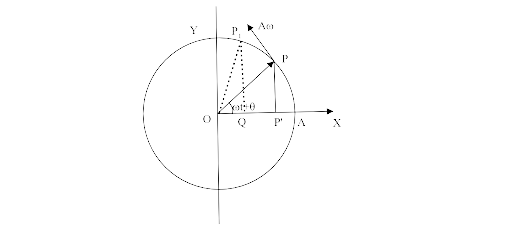Now the particle moves from $P$  to ${P_1}$   then its foot shifts from $P'$  to Q i.e., towards the negative axis. Hence the sign of $\theta$ motion of $P'$  is negative.

14.26. Show that for a particle executing S.H.M, velocity and displacement have a phase difference $\dfrac{\pi }{2}$

Ans: Consider a SHM $x = A\sin \omega t - - - - (i)$

$v = \dfrac{{dx}}{{dt}} = A\omega \cos \omega t$

$= A\omega \sin ({90^0} + \omega t)$

$(\because \sin ({90^0} + \theta ) = \cos \theta )$

$\therefore v = A\omega \sin \left( {\omega t + \dfrac{\pi }{2}} \right)$

Phase of displacement from (i) is $(\omega t)$

Phase of velocity from (ii) is $(\omega t + \dfrac{\pi }{2})$

Hence, the phase difference $= \omega t + \dfrac{\pi }{2} - \omega t$

= $\dfrac{\pi }{2}$

14.27. Draw a graph to show the variation of PE, KE and total energy of a simple harmonic oscillator with displacement.

Ans: Consider a spring of spring constant k in attached to a mass lying on a horizontal frictionless surface. Now the mass m is displaced through a distance A from its mean position. Then it will execute simple harmonic motion. P.E. of mass m at this stretched position $= \dfrac{1}{2}k{A^2}$

At maximum stretch K.E $= 0$ at $x = A$

PE = Total Energy $= \dfrac{1}{2}k{A^2}$  at $x = x \pm A$

Now let mass is at its x displacement from mean position then restoring force acts on particle

$PE = \dfrac{1}{2}k{x^2} = \dfrac{1}{2}m{\omega ^2}{x^2}$

Where $k = m{\omega ^2}$ is called restoring force constant of oscillator when $x = \pm A$ then

$P{E_{\max }} = \dfrac{1}{2}m{\omega ^2}{A^2}$

KE of SHM $= \dfrac{1}{2}m{v^2}$   $(\because {v^2} = {\omega ^2}({A^2} - {x^2}))$

$\therefore KE = \dfrac{1}{2}m{\omega ^2}({A^2} - {x^2})$

When $x = \pm A$ , $KE = 0$

 x K.E P.E T.E 0 $\dfrac{1}{2}m{\omega ^2}{A^2}$ $0$ $\dfrac{1}{2}m{\omega ^2}{A^2}$ +A $0$ $\dfrac{1}{2}m{\omega ^2}{A^2}$ $\dfrac{1}{2}m{\omega ^2}{A^2}$ -A $0$ $\dfrac{1}{2}m{\omega ^2}{A^2}$ $\dfrac{1}{2}m{\omega ^2}{A^2}$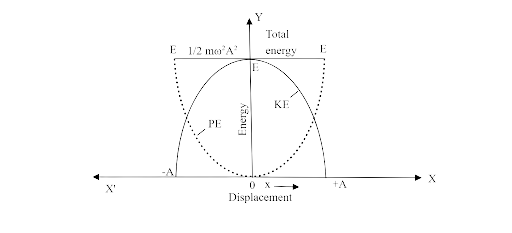Total energy E at any displacement x is given by,

E=PE+KE

$= \;\;\dfrac{1}{2}m{\omega ^2}{x^2} + \dfrac{1}{2}m{\omega ^2}({A^2} - {x^2})$

$= \;\;\dfrac{1}{2}m{\omega ^2}{x^2} + \dfrac{1}{2}m{\omega ^2}{A^2} - \dfrac{1}{2}m{\omega ^2}{x^2}$

$= \dfrac{1}{2}m{\omega ^2}{A^2}$

As E, does not have $x$ in it so it is constant with displacement x.

14.28. Length of a second’s pendulum on the surface of Earth is 1m. What will be the length of a second’s pendulum on the moon?

Ans: A pendulum of time period (T) of 2 sec is called the second pendulum.

${T_e} = 2\pi \sqrt {\dfrac{{{l_e}}}{{{g_e}}}} \Rightarrow {T_e}^2 = 4{\pi ^2}\dfrac{{{l_e}}}{{{g_e}}}......(i)$

$T = 2\pi \sqrt {\dfrac{{{l_m}}}{{{g_m}}}}$    $\because {g_m} = \dfrac{{{g_e}}}{6}$

$\therefore {T_m}^2 = 4{\pi ^2}\dfrac{{{l_m}x6}}{{{g_e}}}.....(ii)$

For second pendulum ${T_e} = {T_m} = 2\sec$

$\dfrac{{{T_m}^2}}{{{T_e}^2}} = \dfrac{{{g_e}}}{{4{\pi ^2}\dfrac{{{l_e}}}{{{g_e}}}}}$   or $\dfrac{{{{(2)}^2}}}{{{{(2)}^2}}} = \dfrac{{6{l_m}}}{{{l_e}}}$

${l_e} = 1m$

$\dfrac{1}{1} = \dfrac{{6{l_m}}}{{1m}} \Rightarrow {l_m} = \dfrac{1}{6}m$

14.29. Find the time period of mass M when displaced from its equilibrium position and then released for the system shown.

Ans: When mass M is pulled and released then mass M oscillates up and down along with pulleys. Let the spring extend by ${x_0}$  when loaded by mass M. The extension and compression of spring from initial position is larger and smaller respectively due to acceleration due to gravity by the same amount of forces always. So the effect of gravitational force can be neglected.Now let the mass ‘M’ be pulled by force ‘F’ downward by displacement x. Then extension in spring will be 2x  as string can not extend. So total extension in spring $= ({x_0} + 2x)$

${T^1} = k({x_0} + 2x)$ (when pulled downwards by x)

$T = k{x_0}$  when no pulling

$F = 2T \Rightarrow F = 2k{x_0}$

And ${F^1} = 2k({x_0} + 2x)$

Restoring force, ${F_{res}} = - (F' - F)$

${f_{rest}} = - [ - 2k(x{}_0 + 2x) - 2k{x_0}]$

${F_{rest}} = - 2k.2x$

$Ma = - 4kx$

$a = - \dfrac{{4k}}{M}x$

$\Rightarrow a$ $\alpha$ $- x$

Hence motion is SHM.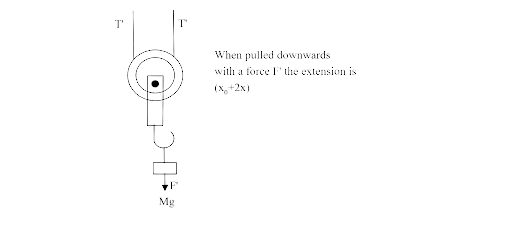$a = - {\omega ^2}x$

$\therefore {\omega ^2} = \dfrac{{ - a}}{x} = \dfrac{{ + 4k}}{M}$

$\omega = 2\sqrt {\dfrac{k}{M}}$

$\Rightarrow \dfrac{{2\pi }}{T} = 2\sqrt {\dfrac{k}{M}}$

$T = \pi \sqrt {\dfrac{M}{k}}$

14.30. Show that the motion of a particle represented by $y = \sin \omega t - \cos \omega t$  is simple harmonic with a period of $\dfrac{{2\pi }}{\omega }$

Ans: A function will represent S.H.M. if it can be written uniquely in the form of  $\sin \left( {\dfrac{{2\pi }}{T}t + \Phi } \right)$

Now $y = \sin \omega t - \cos \omega t$

It is given that, $y = \sqrt 2 \left[ {\sin \omega t\dfrac{1}{{\sqrt 2 }} - \cos \omega t\dfrac{1}{{\sqrt 2 }}} \right]$

$y = \sqrt 2 \left[ {\sin \omega t\cos \dfrac{\pi }{4} - \cos \omega t\sin \dfrac{\pi }{4}} \right]$

$y = \sqrt 2 \sin \left( {\omega t - \dfrac{\pi }{4}} \right)$

Comparing with standard SHM, $y = a\sin (\dfrac{{2\pi }}{T}t + \Phi )$

We get, $\omega = \dfrac{{2\pi }}{T}$ or $T = \dfrac{{2\pi }}{\omega }$

14.31. Find the displacement of a simple harmonic oscillator at which its P.E. is half of the maximum energy of the oscillator.

Ans: Consider an oscillator is at displacement $x$ from its mean position then $PE = \dfrac{1}{2}k{x^2}$,where  $k = m{\omega ^2}$ which is force constant of oscillator of mass m.

$PE = - {m^2}{x^2}$

Maximum potential energy is when kinetic energy is zero, which is when the particle is at its maximum distance from the equilibrium position.

$E = \dfrac{1}{2}m{\omega ^2}{A^2}$

PE is half of the total energy E at displacement$x$ .

$PE = \dfrac{1}{2}Total$$Energy \dfrac{1}{2}m{\omega ^2}{x^2} = \dfrac{1}{2}.\dfrac{1}{2}m{\omega ^2}{A^2} {x^2} = \dfrac{1}{2}{A^2} or x = \pm \dfrac{A}{{\sqrt 2 }} That is, PE will be half of total energy when its displacement is \pm \dfrac{1}{{\sqrt 2 }} amplitude from mean position. 14.32. A body of mass m is situated in a potential field U(x) = {U_0}(1 - \cos \alpha x) where {U_0} and \alpha are constants. Find the time period of small oscillations. Ans: We know that for small work done, dW = F.dx If W = U then, dU = F.dx or F = \dfrac{{ - d{U_x}}}{{dx}} (here restoring force is opposite to displacement). F = \dfrac{{ - d}}{{dx}}\left[ {{U_0}(1 - \cos \alpha x)} \right] = \dfrac{{ - d}}{{dx}}\left[ {{U_0} + {U_0}\cos \alpha x)} \right] F = - \left[ {0 - {U_0}( - \sin \alpha x).\alpha } \right] F = - \alpha {U_0}\sin \alpha x For SHM, \alpha x is small So \sin \alpha x becomes \alpha x - - - - (i) F = - \alpha {U_0}\alpha x = {\alpha ^2}{U_0}x - - - - (ii) \alpha ,{U_0} are constants. \therefore F \propto$$ - x$ , so the motion is SHM

Here from (ii) we get that, $k = {\alpha ^2}{U_0}$

$m{\omega ^2} = {\alpha ^2}{U_0}$

$\Rightarrow {\omega ^2} = {\alpha ^2}\dfrac{{{U_0}}}{m}$

${\left( {\dfrac{{2\pi }}{T}} \right)^2} = {\alpha ^2}\dfrac{{{U_0}}}{m}$

$\Rightarrow {T^2} = 4{\pi ^2}\dfrac{m}{{{U_0}{\alpha ^2}}}$

$T = \dfrac{{2\pi }}{\alpha }\sqrt {\dfrac{m}{{{U_0}}}}$

From (i) this time period is valid for small angles $\alpha x$.

14.33. A mass of 2 kg is attached to the spring of spring constant $50N{M^{ - 1}}$ . The block is pulled to a distance of 5cm from its equilibrium position at x = 0 on a horizontal frictionless surface from rest at t = 0. Write the expression for its displacement at any time t.

Ans: $x{\text{ }} = {\text{ }}5{\text{ }}sin{\text{ }}5t$

14.34. Consider a pair of identical pendulums, which oscillate with equal amplitude independently such that when one pendulum is at its extreme position making an angle of ${2^0}$  to the right with the vertical, the other pendulum makes an angle of ${1^0}$  to the left of the vertical. What is the phase difference between the pendulums?

Ans: ${\theta _1} = {\theta _0}\sin (\omega t + {\delta _1})$

${\theta _2} = {\theta _0}\sin (\omega t + {\delta _2})$

For the first, $\theta = {2^0}$

therefore, $\sin (\omega t + {\delta _1}) = 1$

for the second, $\theta = - {1^0}$

therefore, $\sin (\omega t + {\delta _2}) = - 1$

$\therefore \omega t + {\delta _1} = {90^0}$ , $\omega t + {\delta _2} = - {30^0}$

$\therefore {\delta _1} - {\delta _2} = {120^0}$

14.35. A person normally weighing 50 kg stands on a massless platform which oscillates up and down harmonically at a frequency of $2.0{s^{ - 1}}$ and an amplitude of $5.0\,cm$ . A weighing machine on the platform gives the person's weight against time.

(a) Will there be any change in weight of the body, during the oscillation? Figure Inextensible string.

Ans: Weight in the weight machine will be due to the normal reaction (N) by platform. Consider the top position of the platform, two forces due to weight of the person and the oscillator acts both downward.So motion is downwards, let ‘a’ be the acceleration then,

$ma = mg - N - - - (i)$

When platform lifts form its lowest position to upward

$ma = N - mg - - - - (ii)$

$a = {\omega ^2}A$  is the acceleration of the oscillator.

From (i),

$N = mg - m{\omega ^2}A$

Where $A$  is the amplitude, $\omega$ is the angular frequency, $m$ is the mass of the oscillator.

$\omega = 2\pi v$

$= (2\pi )2$

$= 4\pi \dfrac{rad}{s}$

$A = 5X{10^{ - 2}}m$

$m = 50kg$

$N = 50X9.8 - 50X4\pi X4\pi X5X{10^{ - 2}}$

$= 50[9.8 - 16{\pi ^2}X5X{10^{ - 2}}]$

$= 50[9.8 - 80X3.14X3.14X{10^{ - 2}}]$

$N = 50[9.8 - 7.89]$

$= 95.50N$

So minimum weight is 95.50 N

from  equation (ii) , $N{\text{ }}--{\text{ }}mg{\text{ }} = {\text{ }}ma$

For upward motion form lowest point of oscillator

$N = mg + ma = m(a + g)$

$= m[9.18 + {\omega ^2}A]a = {\omega ^2}A$

$= 50[9.81 + 16{\pi ^2}X5X{10^{ - 2}}]$

$= 50[9.81 + 7.89] = 50[17.70]$

$N = 885.00N$

Hence, there is a change in weight of the body during oscillation.

(b) If the answer to part (a) is yes, what will be the maximum and minimum reading in the machine and at which position?

Ans: The maximum weight is 885 N, when the platform moves from lowest to upward direction. And the minimum is 95.5 N, when the platform moves from the highest point to downward direction.

14.36. A body of mass m is attached to one end of a massless string which is suspended vertically from a fixed point. The mass is held in hand so that the spring is neither stretched or compressed. Suddenly the support of the hand is removed. The lowest position attained by the mass during oscillation is 4cm below the point, where it was held in hand.

(a) What is the amplitude of oscillation?

Ans: When mass m is held in support by hand the extension in spring will be zero as no deforming force acts on spring. Let the mass reach its new position $x$ unit displacement from previous.

Then P.E. of spring or mass = gravitational P.E. lost by man$PE = mgx$

But PE due to spring is $\dfrac{1}{2}k{x^2}$ , where $k = {\omega ^2}A$

$\therefore \dfrac{1}{2}k{x^2} = mgx$

$x = \dfrac{{2mg}}{k} - - - - (i)$

Mean position of spring by block will be when extension is ${x_0}$ then

$F = \pm k{x_0}$

$F = mg$

$\therefore mg = + k{x_0}$  or ${x_0} = \dfrac{{mg}}{k} - - - - (ii)$

From (i) and (ii)

$x = 2\left( {\dfrac{{mg}}{k}} \right) = 2{x_0}$

$x = 4cm$   $\therefore 4 = 2{x_0}$

${x_0} = 2cm$

The amplitude of oscillator is the maximum distance from mean position, that is

$x - {x_0} = 4 - 2 = 2cm$

(b) Find the frequency of oscillation?

Ans: Time period , $T = 2\pi \sqrt {\dfrac{m}{k}}$ , which does not depend on amplitude.

$\dfrac{{2mg}}{k} = x$ , from (i)

$\dfrac{m}{k} = \dfrac{x}{{2g}} = \dfrac{{4X{{10}^{ - 2}}}}{{2X9.8}}$  , this can be written as $\dfrac{k}{m} = \dfrac{{2X9.8}}{{4X{{10}^{ - 2}}}}$

$v = \dfrac{1}{{2\pi }}\sqrt {\dfrac{k}{m}} = \dfrac{1}{{2X3.14}}\sqrt {\dfrac{{2X9.8}}{{4X{{10}^{ - 2}}}}}$ = $\sqrt {\dfrac{{4.9X{{10}^2}}}{{6.28}}}$

$v = \dfrac{{10X2.21}}{{6.28}} = 3.52Hz$

Oscillator will not rise above the positive from where it was released because total extension in spring is 4cm when released and amplitude is 2 cm. So it oscillates below the released position.

14.37. A cylindrical log of wood of height h and area of cross-section A floats in water. It is pressed and then released. Show that the log would execute S.H.M. with a time period $T = 2\pi \sqrt {\dfrac{m}{{A\rho g}}}$, where m is mass of the body and ρ is density of the liquid.

Ans: When log is pressed downward into the liquid then an upward Buoyant force  action which moves the block upward and due to inertia it moves upward from its mean position due to inertia and then again comes down due to gravity. So net restoring force on block = Buoyant force – mgV= volume of liquid displaced by block

When it floats,

mg= buoyant force or $mg = V\rho g$

$mg = A{x_0}\rho g - - - (i)$

A= area of cross section

${x_0} =$ height of block liquid

Let x height again dip in liquid when pressed into water total height of block in water = $(x + {x_0})$

The net restoring force= $[A(x + {x_0})]\rho g - mg$

${F_{restoring}} = A{x_0}\rho g + Ax\rho g - A{x_0}\rho g$

${F_{restoring}} = - Ax\rho g$

(as Buoyant force is upward and x is downward)

${F_{restoring}} \propto - x$

So motion is SHM.

$a = - {\omega ^2}x$ , but ${\omega ^2} = \dfrac{k}{m}$

$\Rightarrow T = 2\pi \sqrt {\dfrac{m}{k}}$

${F_{restoring}} = - A\rho gx$

$ma = - A\rho gx$

$a = \dfrac{{ - A\rho gx}}{m} \Rightarrow - {\omega ^2}x = \dfrac{{ - A\rho gx}}{m}$

${\omega ^2} = \dfrac{{A\rho g}}{m}$

$k = A\rho g$

${\left( {\dfrac{{2\pi }}{T}} \right)^2} = \dfrac{{A\rho g}}{m}$

$\Rightarrow T = 2\pi \sqrt {\dfrac{m}{{A\rho g}}}$

14.38. One end of a V-tube containing mercury is connected to a suction pump and the other end to the atmosphere. The two arms of the tube are inclined to horizontal at an angle of 45° each. A small pressure difference is created between two columns when the suction pump is removed. Will the column of mercury in the V-tube execute simple harmonic motion? Neglect capillary and viscous forces. Find the time period of oscillation.

Ans: Let the liquid column in both columns be at ${h_0}$ heights initially. Now due to pressure difference the liquid columns in A arm pressed by $x$  and in arm B lifts by  $x$  (so difference in vertical height between two levels =$2\;x$ ) Consider an element of liquid of height $dx$  (in tube)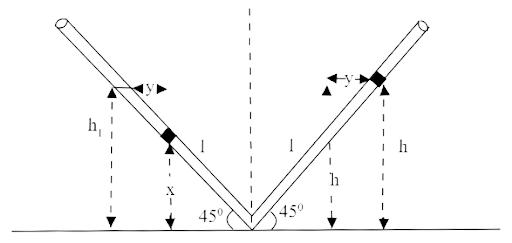Then it’s mass $dm = A.dx.\rho$ , A =area of cross-section of tube

P.E. of the left $dm$  elements column=$(dm)g.h$

P.E. of $dm$ element in left column $= A\rho .g.x.dx$

Total PE in left column $= \int\limits_0^h {A\rho .g.xdx = A\rho g\left[ {\dfrac{{{x^2}}}{2}} \right]} _0^h$

$= A\rho .g\dfrac{{{h_1}^2}}{2}$

From the figure, $\sin {45^0} = \dfrac{{{h_1}}}{l}$

${h_1} = {h_2} = l\sin {45^0} = \dfrac{l}{{\sqrt 2 }}$

${h_1}^2 = {h_2}^2 = \dfrac{{{l^2}}}{2}$

PE in the left column $= A\rho g\dfrac{{{l^2}}}{4}$

Similarly P.E. in right column $= A\rho g\dfrac{{{l^2}}}{4}$

$\therefore$ Total potential energy $= A\rho g\dfrac{{{l^2}}}{4} + A\rho g\dfrac{{{l^2}}}{4} = \dfrac{{A\rho g{l^2}}}{2}$

Due to the pressure difference, let element moves towards the right side is $y$ unit.

Then the liquid column is left arm$= (l - y)$

And the liquid column in right arm$= (l + y)$

P.E. of liquid column in left arm$= A\rho g{(l - y)^2}{\sin ^2}{45^0}$

P.E. of liquid column in right arm $= A\rho g{(l + y)^2}{\sin ^2}{45^0}$

Total P.E. due liquid column$= A\rho g{\left( {\dfrac{1}{{\sqrt 2 }}} \right)^2}\left[ {{{(l - y)}^2} + {{(l + y)}^2}} \right]$

Final P.E. due to difference in liquid columns$= \dfrac{{A\rho g}}{2}\left[ {{l^2} + {y^2} - 2ly + {l^2} + {y^2} + 2ly} \right]$

Final PE= $\dfrac{{A\rho g}}{2}(2{l^2} + 2{y^2})$

Change P.E. = Final P.E. – Initial P.E.

$= \dfrac{{A\rho g}}{2}(2{l^2} + 2{y^2}) - \dfrac{{A\rho g{l^2}}}{2}$

$= \dfrac{{A\rho g}}{2}[2{l^2} + 2{y^2} - {l^2}]$

Change in PE $= \dfrac{{A\rho g}}{2}({l^2} + 2{y^2})$

If change in velocity of total liquid column,

$\Delta KE = \dfrac{1}{2}m{v^2}$

$m = (A.2l)\rho$

$\Delta KE = \dfrac{1}{2}(A.2l.\rho ){v^2} = A\rho l{v^2}$

$\therefore$ Change in total energy $= \dfrac{{A\rho g}}{2}({l^2} + 2{y^2}) + - A\rho l{v^2}$

Total change in energy $\Delta PE + \Delta KE = 0$

$\therefore \dfrac{{A\rho g}}{2}\left[ {{l^2} + 2{y^2}} \right] + A\rho l{v^2} = 0$

$\therefore \dfrac{{A\rho g}}{2}\left[ {g({l^2} + 2{y^2}) + 2l{v^2}} \right] = 0$

$\dfrac{{A\rho }}{2} \ne 0$

$\therefore g({l^2} + 2{y^2}) + 2l{v^2} = 0$

Differentiating with respect to time we get,

$g\left[ {0 + 2X2y\dfrac{{dy}}{{dt}}} \right] + 2l.2v.\dfrac{{dv}}{{dt}} = 0$

$4gy\dfrac{{dy}}{{dt}} + 4vl\dfrac{{{d^2}y}}{{d{t^2}}} = 0$

$4gy.v + 4vl\dfrac{{d{}^2y}}{{d{t^2}}} = 0 \Rightarrow 4v\left[ {gy + l.\dfrac{{{d^2}y}}{{d{t^2}}}} \right] = 0$

$\dfrac{{{d^2}y}}{{d{t^{}}}} + \dfrac{g}{l}y = 0$  because $4v \ne 0$

It is the equation of SHM oscillator and standard equation of SHM is $\dfrac{{{d^2}y}}{{d{t^2}}} + {\omega ^2}y = 0$

${\omega ^2} = \dfrac{g}{l}$

$\dfrac{{2\pi }}{T} = \sqrt {\dfrac{g}{l}} \Rightarrow T = 2\pi \sqrt {\dfrac{l}{g}}$

14.39. A tunnel is dug through the centre of the Earth. Show that a body of mass ‘m’ when dropped from rest from one end of the tunnel will execute simple harmonic motion.

Ans: As the acceleration due to gravity of earth inside the earth is g

$g' = g\left( {1 - \dfrac{d}{R}} \right) = g\left[ {\dfrac{{R - d}}{R}} \right]$

$R - d = y$

$\therefore g' = g\dfrac{y}{R}$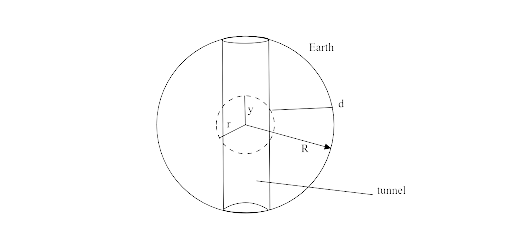Force on both at depth d is,

$F = - mg' = - mg\dfrac{y}{R}$

$F \propto ( - y)$

So the motion of the body in the tunnel is SHM. For a period we can write

$ma = - mg'$

$a = \dfrac{{ - g}}{R}y$

$- {\omega ^2}y = \dfrac{{ - g}}{R}y$

$\dfrac{{2\pi }}{T} = \sqrt {\dfrac{g}{R}}$ or  $T = 2\pi \sqrt {\dfrac{R}{g}}$

14.40. A simple pendulum of time period 1second and length l is hung from a fixed support at O, such that the bob is at a distance H vertically above A on the ground (Figure). The amplitude is $\theta$  . The string snaps at $\theta = \dfrac{{{\theta _0}}}{2}$  . Find the time taken by the bob to hit the ground.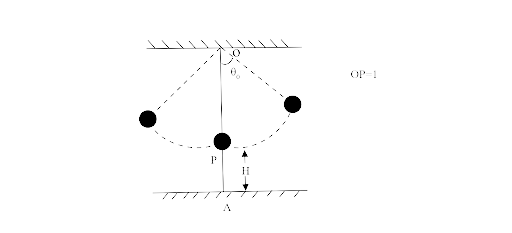Also find distance from A where bob hits the ground. Assume to be small so that $\sin {\theta _0} = {\theta _0}$  and $\cos {\theta _0} = 1$

Ans: Consider the diagram at $t = 0$ ,$\theta = \dfrac{\theta }{2}$

At $t = {t_1}$ , $\theta = \dfrac{{{\theta _0}}}{2}$

$\theta = {\theta _0}\cos \omega t$At $t = {t_1}$ , $\theta = \dfrac{{{\theta _0}}}{2}$

$\therefore \dfrac{{{\theta _0}}}{2} = {\theta _0}\cos \dfrac{{2\pi }}{T}{t_1}$

T=1 sec (given)

$\therefore \cos 2\pi {t_1} = \dfrac{1}{2} = \cos \dfrac{\pi }{3}$

$2\pi {t_1} = \dfrac{\pi }{3}$  or ${t_1} = \dfrac{1}{6}$

$\theta = {\theta _0}\cos 2\pi t$

$\dfrac{{d\theta }}{{dt}} = - {\theta _0}2\pi \sin 2\pi t$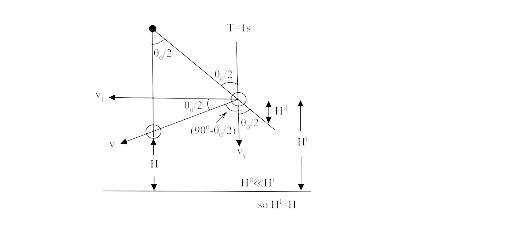At $t = \dfrac{1}{6}$ , ie at $\theta = \dfrac{{{\theta _0}}}{2}$

$\dfrac{{d\theta }}{{dt}} = - {\theta _0}2\pi \sin 2\pi \dfrac{1}{6}$

$\dfrac{{d\theta }}{{dt}} = - {\theta _0}2\pi \sin \dfrac{\pi }{3} = - {\theta _0}2\pi \dfrac{{\sqrt 3 }}{2}$

$\dfrac{{d\theta }}{{dt}} = - {\theta _0}\pi \sqrt 3$

$\omega = - {\theta _0}\pi \sqrt 3$

$v = - \sqrt 3 \pi {\theta _0}l$

(-) ve shows that bob’s motion is towards the left.

${v_x} = v\cos \dfrac{{{\theta _0}}}{2} = - \sqrt {3\pi } {\theta _0}l\sin \dfrac{{{\theta _0}}}{2}$

Let vertical distance covered by ${v_y}$  is $H'$  (downward)

$s = ut + \dfrac{1}{2}g{t^2}$

$H' = {v_y}t + \dfrac{1}{2}g{t^2}$

$\dfrac{1}{2}g{t^2} + \sqrt 3 \pi {\theta _0}l\sin \dfrac{{{\theta _0}}}{2}Xt - H' = 0$

$\sin \dfrac{{{\theta _0}}}{2} = \dfrac{{{\theta _0}}}{2}$    (given)

$\dfrac{1}{2}g{t^2} + \sqrt 3 \pi {\theta _0}l\dfrac{{{\theta _0}}}{2}t - H' = 0$

$\dfrac{1}{2}g{t^2} + \sqrt 3 \pi {\theta _0}l\dfrac{{{\theta _0}^2}}{2}lt - H' = 0$

We know that by quadratic formula, $t = \dfrac{{ - b \pm \sqrt {{b^2} - 4ac} }}{{2a}}$

$t = \dfrac{{( - \sqrt 3 \pi {\theta _0}^2l)/4 \pm \sqrt {(3{\pi ^2}{\theta _0}^4{l^2})/4 + 4.\dfrac{1}{2}gH'} }}{{2g/2}}$

As ${\theta _0}$  is very small so by neglecting ${\theta _0}^4$ and ${\theta _0}^2$

$t = \dfrac{{ + \sqrt {2gH'} }}{g}$ , $H' = H + H''$ , from figure.

$\therefore t = \sqrt {\dfrac{{2H}}{g}} H'' \ll H'$ as $\dfrac{{{\theta _0}}}{2}$ is very small.

$\therefore H = H'$

Distance covered in horizontal $= {v_x}t$

$X = \sqrt 3 \pi {\theta _0}l\cos \dfrac{{{\theta _0}}}{2}\sqrt {\dfrac{{2H}}{g}}$

$\therefore X = \sqrt 3 \pi {\theta _0}l\sqrt {\dfrac{{2H}}{g}}$ ,    ($\because \cos \dfrac{{{\theta _0}}}{2} = 1$ given)

$X = {\theta _0}l\pi \sqrt {\dfrac{{6H}}{g}}$

At the time of snapping, the bob was at distance $l\sin \dfrac{{{\theta _0}}}{2} = l\dfrac{{{\theta _0}}}{2}$  from A

Thus the distance of bob from A where it meet the ground is

$= \dfrac{{l{\theta _0}}}{2} - X = \dfrac{{l{\theta _0}}}{2} - {\theta _0}l\pi \sqrt {\dfrac{{6H}}{g}}$

$= {\theta _0}l\left[ {\dfrac{1}{2} - \pi \sqrt {\dfrac{{6H}}{g}} } \right]$

### About Chapter 14- Oscillations of Class 11 Physics

Oscillation is defined as a repeating variation in a given period of time from the start of a point of equilibrium. Simple harmonic, damped and driven Oscillations, coupled Oscillations, and waves are some of the different forms of Oscillations.

A simple harmonic motion is a sort of periodic oscillatory motion that is introduced in NCERT Exemplar Solutions for Class 11 Physics  Chapter 14. You'll also answer problems involving periodic motion, oscillatory motion, frequency, phase, simple pendulum, damped and undamped simple harmonic motion, and simple harmonic motion characteristics. These NCERT Exemplar Class 11 Physics  for Chapter 14 of Class 11 Physics  have been created by Vedantu highly experienced teachers with your comprehension level in mind.

'Oscillations' is a fascinating Chapter that introduces new ideas, technical terms, project work, and demonstrations. The Chapter has around 5 exercises with a total of 40 sample problems. You may simply get solutions to all of the Chapter's exercises at Vedantu. To clear your notions about Oscillations, put in a lot of practice time.

Here you'll get 100% authentic NCERT Exemplar Class 11 Physics  Problems and Solutions for Chapter 14. According to CBSE requirements, we provide solutions to the questions in the Example textbook. Furthermore, the solutions have been written in such a way that they will meet all of your demands as you study the Chapter. Now is your chance to join for free.

Oscillation is a Chapter in the NCERT Exemplar Class 11 Physics  Solutions that is frequently questioned in Exams. Students will learn about a body's basic harmonic and uniform motion, as well as several other words connected to a body's periodic motion. Different types of Oscillations and waves, as well as their various features, are discussed in NCERT Exemplar Class 11 Physics  Chapter 14 Solutions. NCERT Exemplar Class 11 Physics  Solutions Chapter 14 PDF download can also be used for future reference. This Chapter of the NCERT Class 11 Physics  Syllabus contains various relationships and equations as well as problems that must be learned.

### The Oscillation has a Wide Range of Applications and Uses in the Actual World, Including:

• Fork for tuning

• String that vibrates

• Vibration in the torsional plane

### Oscillations are an Important Topic in Class 11 Physics  Chapter 14.

• Oscillatory and periodic motions

• Harmonic motion in its most basic form

• Harmonic motion is simple and circular motion is uniform.

• In basic harmonic motion, velocity and acceleration are measured.

• For simple harmonic motion, there is a force law.

• Simple harmonic motion of energy

• SHM Ex is being executed on some computers.

• Simple harmonic motion that has been dampened

• Forced Oscillations and resonance are two types of forced Oscillations.

### NCERT Physics  Exemplar Solutions Class 11 Chapter 14 – Oscillations: Exercise-by-Exercise Discussion

#### Multiple Choice Questions I –  (Exercise 14.1)

There are 11 multiple-choice exemplar problems in Chapter 14 – Oscillations' first exercise. Explanatory visuals are included with some of these questions to help you understand them better.

#### Multiple Choice Questions II (Exercise 14.2)

There are additional multiple-choice exemplar problems in this drill. There are a total of seven questions that will help you understand the Chapter's themes.

#### Exercise 14.3 - Questions with Very Short Answers

There are ten exemplar problems in Exercise 14.3 of NCERT Exemplar Class 11 Physics  Chapter 14. These are questions with very brief answers based on a form of periodic oscillatory motion known as a simple harmonic motion.

#### Short Answer Type Questions (Exercise 14.4)

This activity includes six short answer type sample problems on periodic motion, oscillatory motion, frequency, phase, simple pendulum, damped and undamped simple harmonic motion, and simple harmonic motion characteristics.

## FAQs on NCERT Exemplar for Class 11 Physics Chapter 14 - Oscillations (Book Solutions)

1. Is NCERT Exemplar for Class 11 Physics  Chapter 14 - Oscillations (Book Solutions) useful?

Oscillations in NCERT Exemplar Class 11 Physics  is a very important study material for CBSE Class 11 students. This Chapter covers the fundamental ideas needed to comprehend some of the topics covered in CBSE Class 12. The NCERT exemplar Class 11 Physics  Chapter 14 PDF has essential questions on Oscillations, numerical on Oscillations, MCQs, Short and long answer questions, important questions from previous year question papers, and sample papers on Oscillations. Vedantu helps students to understand the NCERT solutions in a simpler and easy to understand manner. You can download the PDF from the website.

2. How important is studying Oscillations in Class 11th?

Different types of Oscillations and waves, as well as their various features, are discussed in NCERT Exemplar Class 11 Physics  Chapter 14 Solutions. NCERT Exemplar Class 11 Physics  Solutions Chapter 14 PDF download can also be used for future reference. This Chapter of the NCERT Class 11 Physics  Syllabus contains various relationships and equations as well as problems that must be learned. Download the free PDF from Vedantu now. Physics  is an important discipline to study. Oscillations are used in daily life applications and hence the students should get thorough knowledge for the same.

3. Are the answers in NCERT Exemplar for Class 11 Physics  Chapter 14 - Oscillations (Book Solutions)  authentic?

Here you'll get 100% authentic NCERT Exemplar Class 11 Physics  Problems and Solutions for Chapter 14. According to CBSE requirements, we provide solutions to the questions in the Example textbook. Furthermore, the solutions have been written in such a way that they will meet all of your demands as you study the Chapter. Now is your chance to join Vedantu for free and study with professionals. All the questions are solved by well-read professionals at Vedantu. Hence the authenticity of the website is legit.

4. Is studying Physics  from Vedantu beneficial?

These NCERT Exemplar Class 11 Physics  for Chapter 14 of Class 11 Physics  have been created by Vedantu highly experienced teachers with your comprehension level in mind. 'Oscillations' is a fascinating Chapter that introduces new ideas, technical terms, project work, and demonstrations. The Chapter has around 5 exercises with a total of 40 sample problems. You can get solutions to all of the Chapter's exercises at Vedantu. Apart from these, there are many benefits. You can get other guidebooks and easy to read question-answers from the same. Register now.

5. Can I prepare for JEE with NCERT Exemplar for Class 11 Physics  Chapter 14 - Oscillations (Book Solutions)?

NCERT exemplar Class 11 Physics  Chapter 14 PDF has essential questions on Oscillations, numerical on Oscillations, MCQs, Short and long answer questions, important questions from previous year question papers, and sample papers on Oscillations. Oscillation is defined as a repeating variation in a given period of time from the start of a point of equilibrium. Vedantu can hence help you prepare for JEE mains. It requires dedication and practice along with the proper coaching and guidance from Vedantu. They can help you get prepared for basic fundamentals of Physics  and hence prove to be helpful in JEE.